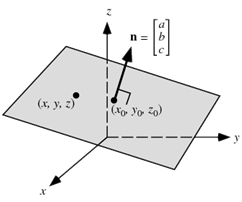# The direction ratios of normal to the plane through the points (0,-1,0) and (0,0,1) and making an angle   with the plane  are :Option 1)  Option 2)Option 3)  Option 4)

Cartesian equation of plane passing through a given point and normal to a given vector -

- whereinPutting in

We get

Angle between two planes (Cartesian form) -

Let the two planes be

then the angle between them is defined as the angle between their normals

-

Equation of line passing through  &   is

Now,

So direction ratios :

So answer are (1) and (3)

Option 1)

Option 2)

Option 3)

Option 4)

Exams
Articles
Questions